Understanding Under Sampling/Aliasing Methods in High-Speed Digitizers/Oscilloscopes

Overview

This tutorial recommends tips and techniques for using National Instruments high-speed digitizers to build the most effective data sampling system possible. In this tutorial, you will learn fundamental information about the underlying theory of sampling with a high-speed digitizer and various methods to optimize the performance of your data sampling. This section of the tutorial covers the topics below.

Real-Time Sampling

Real-time sampling is the default sampling method used by NI high-speed digitizers. In real-time sampling, the digitizer gathers all the samples for a waveform using an internal or external sample clock.

Real-time sampling is the only sampling method available when the signal being sampled is not repetitive.

Equivalent Time Sampling

Equivalent-time sampling (ETS) is a sampling method in which a picture of a waveform is created over time by using a series of samples taken from repetitive waveforms.

Random Interleaved Sampling (RIS) is a form of equivalent-time sampling that increases apparent sample rates of repetitive signals by combining several triggered waveforms. Because the trigger time occurs randomly between two samples, the digitizer samples different points in the waveform on consecutive acquisitions. By combining these waveforms, you can achieve RIS sample rates that are up to 25 times higher than the ADC sample rate on the digitizer.

How RIS Works

Each waveform trigger occurs at some time randomly distributed between two samples. The digitizer can measure the time from the trigger to the next sample, commonly called the time-to-digital conversion (TDC), extremely accurately—with hundreds of times more resolution than the sample period of the digitizer. With these TDC measurements, NI-SCOPE can combine multiple waveforms, aligned at the trigger time, to simulate a faster sampling rate for repetitive signals.

How Oversampling Factors Increase Effective Sample Rates

When a digitizer is in RIS mode, the valid sample rates become multiples of the maximum real-time sampling rate. These multiples are the oversampling factor. For example, the NI 5112 has a 100 MS/s maximum real-time sampling rate, so the RIS sampling rates are the oversampling factor times 100 MS/s, where the oversampling factor is two, three, four, and so on. If you specify 300 MS/s for your sampling rate, the oversampling factor is three.

Each TDC value is between zero and the sampling period, so a 100 MS/s digitizer has TDC values between 0 and 10 ns. This time span is divided into a number of bins equal to the oversampling factor. For example, an oversampling factor of three means there is one TDC bin from 0 to 3.33 ns, another from 3.33 to 6.67 ns, and another from 6.67 to 10 ns. To reconstruct a waveform, each bin must contain at least one TDC value.

The following four figures show an example RIS acquisition with an oversampling factor of three. Each of the figures shows the aligned trigger time of the waveforms followed by the three TDC bins, where the combined width of the three bins is the real-time sample period of the digitizer.

1. The first waveform has a TDC value that falls in bin number 1.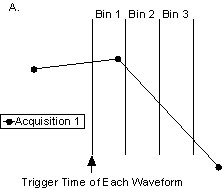2. The succeeding waveform falls in bin number 3.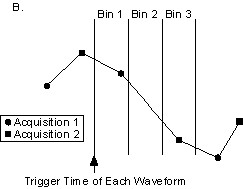3. Because of the randomness of the TDC value, another sampled waveform falls in bin 1. NI-SCOPE does not fetch this waveform from the digitizer because the bin is already filled.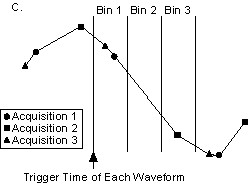4. The next waveform falls in bin 2.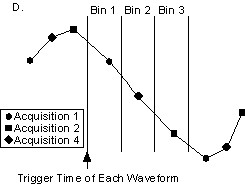Now all bins are full, so NI-SCOPE returns the three waveforms sampled at the maximum real-time rate as one evenly-sampled waveform with a sampling rate three times greater.

Averaging to Minimize Noise

At a minimum to complete an RIS acquisition, the number of TDC values must be equal to the oversampling factor. However, this does not guarantee a cleanly reconstructed signal. The following figures show three acquisitions of a perfectly linear edge.

The following figure shows that the sample in bin number 1 is extremely close to its right bin edge, while the sample in bin number 2 is near its left edge. When NI-SCOPE returns the RIS waveform, the samples are coerced to the middle of each bin to produce an evenly sampled waveform.The following figure shows how NI-SCOPE returns the RIS waveform, with evenly-spaced samples.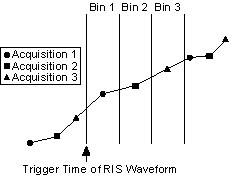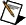Note   You can avoid noise in RIS waveforms by averaging multiple waveforms in each bin during the RIS algorithm. The number of averages in each bin can be specified with the RIS Num Averages attribute of NI-SCOPE.

The following figure shows the acquisition using two averages. Notice that twice as many waveforms are required now.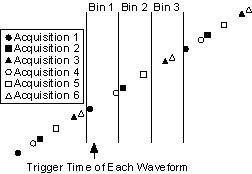The following figure shows the returned waveform from the averaged RIS acquisition.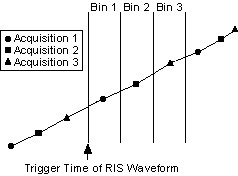The higher the number of averages, the smaller the effect of coercing the time of samples. Therefore, it is generally important to use some amount of averaging with RIS. Increasing the amount of averaging increases the minimum number of waveforms necessary to reconstruct the RIS waveform and therefore, the time it takes to complete an acquisition.

Randomness in RIS Acquisitions

When using RIS, remember that the TDC value is completely random—the hardware does not adjust anything to ensure an even distribution of TDC values. If the TDC is the same from two acquisitions, the digitizer has effectively sampled the same places on the repetitive waveform, so no information has been gained. Therefore, for RIS to work, you must have a suitable random distribution of TDC values, which requires a nondeterministic number of waveforms. While it can take a long time to receive a suitable set of waveforms with different TDC values, in practice you rarely need to wait long. Furthermore, NI-SCOPE employs multiple-record acquisitions and double buffering to optimize reconstructing the waveform while the acquisition is in progress.Note   Sometimes a RIS acquisition times out and does not complete. If increasing the timeout value does not solve the problem, you may need to self-calibrate the digitizer. This timeout can also occur if the digitizer is frequency locked to the same reference as the frequency source.